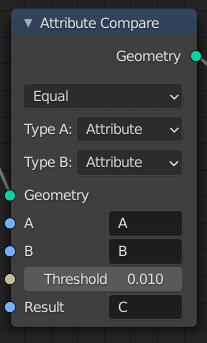# Attribute Compare¶The Attribute Compare node.

This node reads two input attributes as floats and allows for basic elementwise comparison operations. Like the Attribute Math node, it is also possible to switch to using single values as inputs. This node can be combined with the Point Separate node for more control over which points to separate to the second output geometry.

## Inputs¶

Geometry

Standard geometry input.

A, B

The first and second input to the math operation. Depending on the Type input, this is either an attribute name or an input of the specified data type.

Threshold

This value is used as a threshold for still considering the two inputs as equal for the Equal and Not Equal operations.

Result

Name of the attribute where the computed result it stored. If an attribute with this name does not exist yet, a new attribute with a Boolean data type is added. If it does exist, the values of the existing attribute are overridden.

## Properties¶

Operation

The math function to apply.

Note

For operations besides Equal and Not Equal, the input attributes are converted implicitly to the float data type. For the equality operations on vectors, the distance between the vector inputs is used.

Type A, B

Controls whether to use an attribute or a single value of the specified data type as an input.

## Output¶

Geometry

Standard geometry output.